Normal view MARC view ISBD view

# An introduction to the mathematical theory of waves / Roger Knobel.

Material type:TextSeries: Student mathematical libraryIAS/Park City mathematical subseries: v. 3Publisher: 2000Edition: first indian edition 2012.Description: xiv, 196 p. : ill. ; 22 cm.ISBN: 9780821887295 (pbk.) :.Subject(s): Wave-motion, Theory ofDDC classification: 531.1133 Summary: Gifted by NBHM.
Item type Current location Call number Status Date due Barcode Item holds
Book Chennai Mathematical Institute
General Stacks
531.113 KNO (Browse shelf) Available 8459
Total holds: 0
##### Browsing Chennai Mathematical Institute Shelves , Shelving location: General Stacks Close shelf browser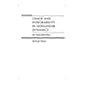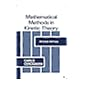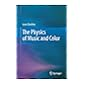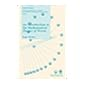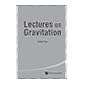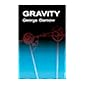531.11 TOB Chaos and integrability in nonlinear dynamics : an introduction / 531.113 CER Mathematical methods in kinetic theory / 531.113 GUN The physics of music and color / 531.113 KNO An introduction to the mathematical theory of waves / 531.113 LEI Nonlinear waves / 531.14 DAS Lectures on gravitation / 531.14 GAM Gravity /

Includes bibliographical references and index.